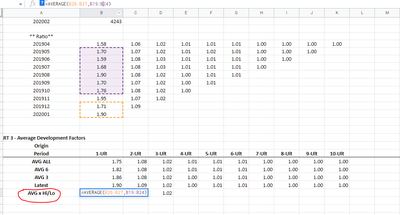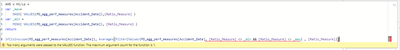cancel
Showing results for
Did you mean:Helper I

## Average without the highest and lowest number of a measure! Help!

Hi, I am reposting because I need help with this.

I am struggling to find a way to find a measure to calculate the average EXCLUDING THE HIGHEST AND LOWEST numbers from my ratio_measure. Please see the data in excel with the formulas of what I want to accomplish and the BI dashboard here: https://drive.google.com/drive/folders/1tw2kxHBZ93OjckLULVxbonYXQaWjPDld?usp=sharing

When you open the link above you will see the excel sheet with the formulas I am trying to accomplish. The Ratio_Measure is a division between the previous DEV_MONTH/ Most current DEV_MONTH:The expected output will be just like this picture below:* Please see formulas on the excel sheet*What is the best way to accomplish this as MAX and MIN don't accept a measure?

Any help is very much appreciated.

Grateful for this community!

Michelle.

2 REPLIES 2Super User

measure =

var _max = maxx(values(Table[Period]), [Meausre])

var _min= maxx(values(Table[Period]), [Meausre])

return

if(isinscope(Table[Period]), [Measure], Averagex(filter(Values(Table[Period]), [Measure] <> _min && [Measure] <> _max) , [Measure]))

Measure is the current measure you are usingHelper I

Hi @amitchandak ,

Thanks for the help but I keep getting this error below:Thanks,
Michelle